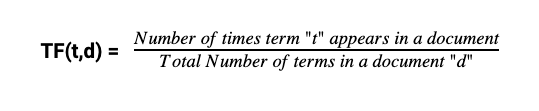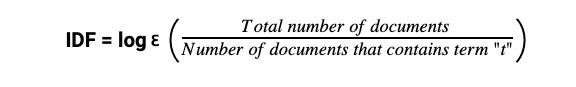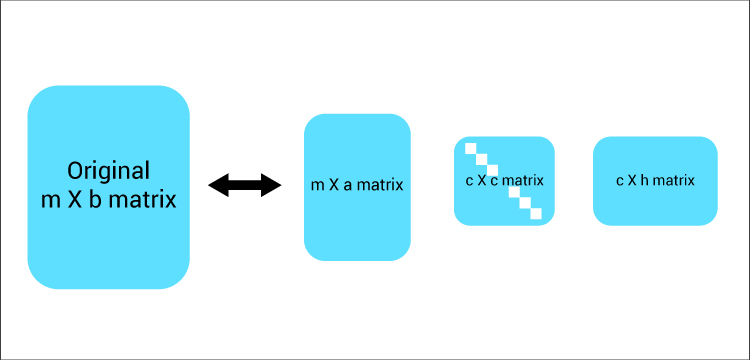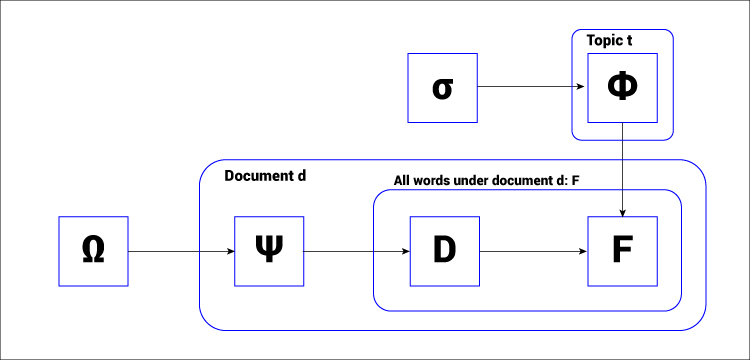• Category
• >NLP

# Introduction of Latent Semantic Analysis (LSA) and Latent Dirichlet Allocation (LDA)

• Tanesh Balodi
• Oct 22, 2019A brilliant scientist once quoted that became fundamentals of natural language processing which is:

"Computers are incredibly fast, accurate and stupid; humans are incredibly slow, inaccurate and brilliant; together they are powerful beyond imagination." - Albert Einstein

Although new word embedding technique which is known to be a state-of-the-art natural language processing technique is able to perform several NLP tasks all at one model but before these models came and changed the game forever we had effective approaches for information retrieval and other NLP problems, two of these approaches include Latent Semantic Analysis (LSA) and Latent Dirichlet Allocation(LDA), both of these methods perform different tasks and were widely used, LSA was introduced in 2005 whereas LDA was introduced in 2003 and became one of the most powerful techniques for text classification and summarization, we are going to discuss about its working and application in detail.

## What’s Inside?

1. Latent Semantic Analysis

2. Term Frequency/Inverse Document Clustering

3. Singular Value Decomposition

4. Latent Dirichlet Allocation

5. Application of LDA

6. Conclusion

## Latent Semantic Analysis(LSA)

Latent Semantic Analysis is one of the natural language processing techniques for analysis of semantics, which in broad level means that we are trying to dig out some meaning out of a corpus of text with the help of statistical and was introduced by Jerome Bellegarde in 2005.

LSA is basically a technique where we identify the patterns from the text document or in simple words we tend to find out relevant and important information from the text document. If we talk about whether it is supervised or unsupervised way, it is clearly an unsupervised approach.

It is a very helpful technique in the reduction of dimensions of the matrix or topic modeling and is also known as Latent Semantic Indexing(LSI). The main concept and work of LSA are to group together all the words that have a similar meaning.

So how does it work? Let’s see ->

### Significance of Term Frequency/ Inverse Document Frequency in LSA

• Term Frequency is defined as a number of times instance or keyword appears in a single document divided by the total number of words in that document.As we know the length of the document is different in each case, so term frequency varies with the occurrence of term respectively.

• Inverse Document Frequency(IDF), signifies how important the term is to be in the collection of documents. IDF calculates the weight of rare term of the text in a collection of documents. The formula of IDF is given byThe main idea of Tf/IDF in Latent Semantic Analysis is to provide each word count and the frequency of rare words in order to provide them weights on the basis of their rarity, TF/IDF is more preferable than conventional counting of occurrence of the word as it only counts the frequency without classification.

After we have done the classification part using TF/IDF we tend to move to our next step that is the reduction of matrix dimension as normally with so many features the input have higher dimensions, a higher dimension input is hard to understand and interpret, so to lower the dimension with maximum information gain we have many techniques which include Singular Value Decomposition(SVD) and Principal Component Analysis. Let’s see what SVD would do after our first step:-Singular value decomposition is a method for matrix decomposition from higher to lower, it usually divides the matrix into three matrices. Let us take an input matrix m x b of higher dimension as ‘A’, to calculate the SVD we will use the formula given below

A(m x b) = U(m x m). σ VT

Here, σ is a diagonal matrix of size m x n and VT is a transpose of n x n orthogonal matrix. SVD may perform several other tasks but remains efficient primarily for dimension reduction, it is widely used and accepted by machine learning developers.

Whenever SVD is performed, results are always classy, it can dramatically reduce more than 150k parameters or dimension to an understandable 50 to 70 parameters. With the completion of the above two tasks, it fulfills the motive of latent semantic analysis.There is much application of LSA to perform but it is mainly used in search engines as it is a very helpful technique there, for example, you searched ‘sports’ and the results also showed cricket and cricketers, this is due to LSA being applied on the search engines. Other possible applications of LSA are document clustering in text analysis, recommender systems and building user profiles.

## Latent Dirichlet Allocation(LDA)

Latent Dirichlet Allocation(LDA) uses Dirichlet distribution(no wonder why it is named latent Dirichlet allocation), So what is Dirichlet distribution? It is a probability distribution but is much different than the normal distribution which includes mean and variance, unlike the normal distribution it is basically the sum of probabilities which combine together and added to be 1.

It has distinct K values for the number of k means the number of probabilities needed for example:

0.6 + 0.4 = 1 (k=2)

0.3 + 0.5 + 0.2 = 1 (k=3)

0.4 + 0.2 + 0.3 + 0.1 = 1 (k=4)

So we can list probabilities as categories which is one of the prominent reasons it is also known as categorical distribution. But how does this probability distribution helps in the method? Let’s see

Let us take a sentence to make a clear illustration of what LDA does exactly,

1. I love cricket.

2. Virat Kohli is my favorite cricketer.

3. The mountains are so beautiful.

4. I would like to visit the Himalayas.

Above are the sentences that are tokenized from different documents, now what LDA does is, it will form clusters or group sentence 1 and 2 together as it has the same contextual meaning and 3 and 4 together to show similarities between the documents or sentences. I hope you got the idea behind LDA, now quickly begin its working:Ω is the topic distribution on per document

Ψ is the distribution for document d

D is the topic for the nth word in a document

F is the chosen specific word

Φ is the word distribution for topic t

σ is the probability of each topic

Above is the working of LDA as we can observe all the probabilities are Dirichlet distribution, While performing LDA or other text summarization method, we tend to remove all the factors that have no relevance, there is a method through which we can remove stop words like “the”, “are”, “is”, “with” etc. these stop words hold no value for document clustering and needs to be removed.

LDA was introduced in 2003 by David Blei, Andrew Ng, and Michael I. Jordan and is also a type of unsupervised learning as LSA. It also has the LDA2vec model in order to predict the other word in sequence same as word2vec, so it becomes an effective technique in the next word prediction.

### Applications Of LDA

1. Phenomenal results on a massive dataset of Gensim, VW and mallet which lead towards great accuracy.

2. Finding patterns that relate or distinguish the scenarios, or in general, helps in pattern recognition in between two documents.

3. Much of the research in topic modeling is done with the help of Dirichlet distribution, which also helped in developing some new algorithms.

4. One of its applications also includes network analysis, which includes network pattern analysis and assortative network mixing analysis.

## Conclusion

Although there are a bunch of NLP techniques available which can perform way better on larger dataset, I personally believe that for beginners, conventional NLP methods are better because they perform better on smaller dataset and are easy to implement, so all the beginners should try to implement these text summarization technique and should move forward slowly and gracefully.  For more blogs in Analytics, machine learning, deep learning, artificial intelligence, natural language processing, and new technologies do read Analytics Steps.